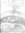# Mathamania discussion

10 views
History of math

message 1: by Joon, Math genius (new)

In this discussion, we'll talk all about the history of math and how our world revolves around it, and all that kind of stuff. First one: How was the theory of Pi started?

message 2: by (new)

message 3: by Joon, Math genius (new)

You can use any source necessary to answer the question.

message 4: by (new)

message 5: by Joon, Math genius (new)

Nope. I'm trying to keep this group alive.

message 6: by (new)Yeah, this group is sort of dying. No! I don't want it to die! Let's keep it alive!

Cool questions, I'll have to look it up though.

message 7: by Joon, Math genius (new)

message 8: by (last edited Apr 06, 2009 10:32AM) (new)There is really no simple answer to this question as there is no definite "discovery" or "discoverer" of the Theory of Pi. Here are some bits and pieces from Wikipedia's article on Pi:

The history of π parallels the development of mathematics as a whole. Some authors divide progress into three periods: the ancient period during which π was studied geometrically, the classical era following the development of calculus in Europe around the 17th century, and the age of digital computers.

Geometrical period

That the ratio of the circumference to the diameter of a circle is the same for all circles, and that it is slightly more than 3, was known to ancient Egyptian, Babylonian, Indian and Greek geometers. The earliest known approximations date from around 1900 BC; they are 25/8 (Babylonia) and 256/81 (Egypt), both within 1% of the true value....Around AD 265, the Wei Kingdom mathematician Liu Hui provided a simple and rigorous iterative algorithm to calculate π to any degree of accuracy. He himself carried through the calculation to a 3072-gon and obtained an approximate value for π of 3.1416....Around 480, the Chinese mathematician Zu Chongzhi demonstrated that π ≈ 355/113, and showed that 3.1415926 < π < 3.1415927 using Liu Hui's algorithm applied to a 12288-gon. This value was the most accurate approximation of π available for the next 900 years....

Classical period

Until the second millennium, π was known to fewer than 10 decimal digits. The next major advance in π studies came with the development of calculus, and in particular the discovery of infinite series which in principle permit calculating π to any desired accuracy by adding sufficiently many terms....Madhava was able to calculate π as 3.14159265359, correct to 11 decimal places....The record was beaten in 1424 by the Persian mathematician, Jamshīd al-Kāshī, who determined 16 decimals of π....The first major European contribution since Archimedes was made by the German mathematician Ludolph van Ceulen (1540–1610), who used a geometric method to compute 35 decimals of π. He was so proud of the calculation, which required the greater part of his life, that he had the digits engraved into his tombstone....Isaac Newton himself derived a series for π and calculated 15 digits, although he later confessed: "I am ashamed to tell you to how many figures I carried these computations, having no other business at the time." In 1706 John Machin was the first to compute 100 decimals of π,...A remarkable record was set by the calculating prodigy Zacharias Dase, who in 1844 employed a Machin-like formula to calculate 200 decimals of π in his head at the behest of Gauss. The best value at the end of the 19th century was due to William Shanks, who took 15 years to calculate π with 707 digits, although due to a mistake only the first 527 were correct. (To avoid such errors, modern record calculations of any kind are often performed twice, with two different formulas. If the results are the same, they are likely to be correct.)...Theoretical advances in the 18th century led to insights about π's nature that could not be achieved through numerical calculation alone. Johann Heinrich Lambert proved the irrationality of π in 1761, and Adrien-Marie Legendre also proved in 1794 π2 to be irrational....

Computation in the computer age

The advent of digital computers in the 20th century led to an increased rate of new π calculation records. John von Neumann used ENIAC to compute 2037 digits of π in 1949, a calculation that took 70 hours. Additional thousands of decimal places were obtained in the following decades, with the million-digit milestone passed in 1973. Progress was not only due to faster hardware, but also new algorithms....In the beginning of the 20th century, the Indian mathematician Srinivasa Ramanujan found many new formulas for π, some remarkable for their elegance and mathematical depth....The Chudnovsky brothers used this formula to set several π computing records in the end of the 1980s, including the first calculation of over one billion (1,011,196,691) decimals in 1989....used by Yasumasa Kanada and team to set most of the π calculation records since 1980, up to a calculation of 206,158,430,000 decimals of π in 1999. The current record is 1,241,100,000,000 decimals, set by Kanada and team in 2002....

And as to the symbol:
The Greek letter π, often spelled out pi in text, was adopted for the number from the Greek word for perimeter "περίμετρος", first by William Jones in 1707, and popularized by Leonhard Euler in 1737.

Well, there's a bit anyway. Sorry it's kind of long.